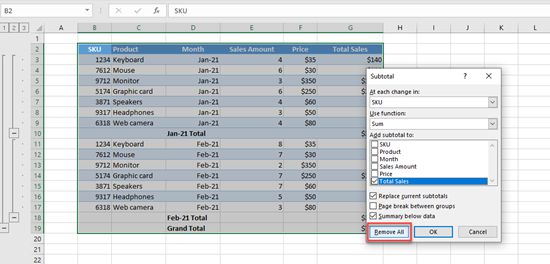# How to Add a Total or Subtotal Row to a Table in Excel

In this article, you will learn how to add a total or subtotal row to a table in Excel.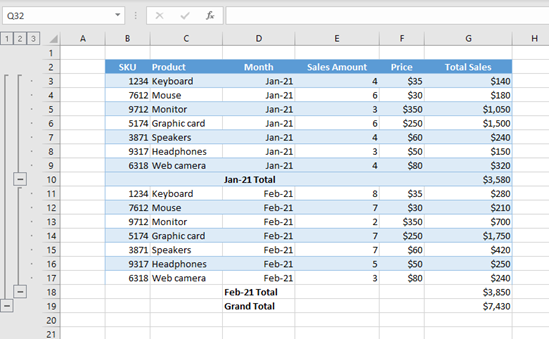If we have a table in Excel with numeric data, we can easily add a total row to it. For example, say that we have a table with products, prices, and sales by month.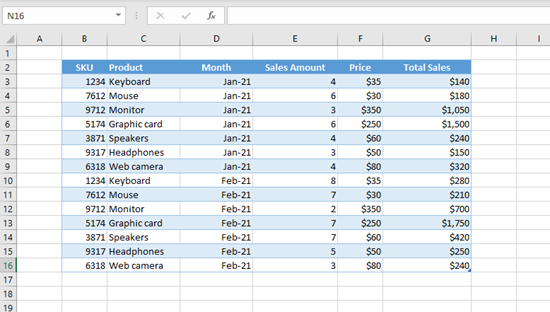We want to add a total for Column G (Total Sales). In order to achieve this, we need to (1) click anywhere in the table. After that, in the Ribbon, Table Design appears. (2) Click on Table Design, then (3) check Total Row. A new row is added at the end of the table with the total amount of sales revenue.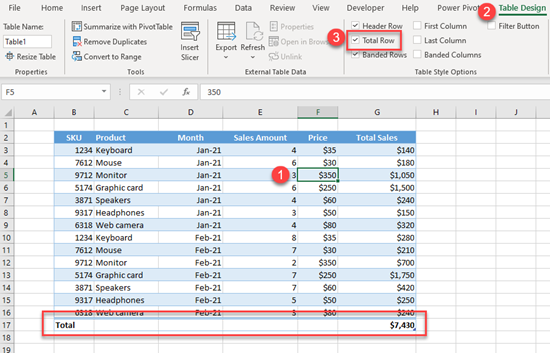We could also insert the total row with a keyboard shortcut: CTRL + SHIFT + T.

If we want to remove totals, we need to uncheck Total Row in the Table Design tab or again use the shortcut CTRL + SHIFT + T.

By default, we can’t insert subtotal rows to an Excel table, but we can do it if we convert the table to a data range. To do this, we need to (1) click anywhere in the table, then in the Ribbon, (2) go to the Table Design tab, and (3) click on Convert to Range.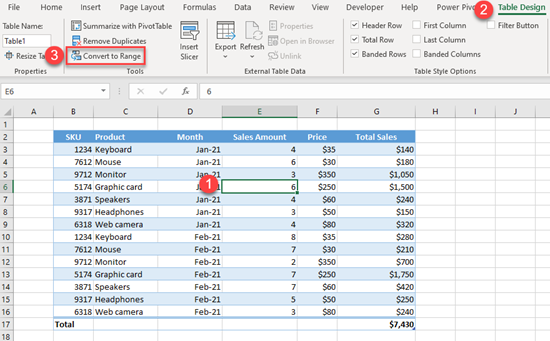We have the same data, but now it’s a normal range instead of an Excel table. To check this, click anywhere in the data, and we’ll see that Table Design tab doesn’t appear in the Ribbon.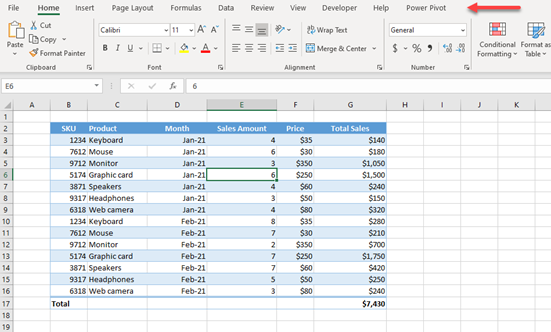Now we can add subtotal rows to our data range. Let’s say that we want to group data and add subtotals by month (Column D). First, we need to (1) click anywhere in the data, then in the Ribbon, (2) go to the Data tab, and in Outline (3) click on Subtotal.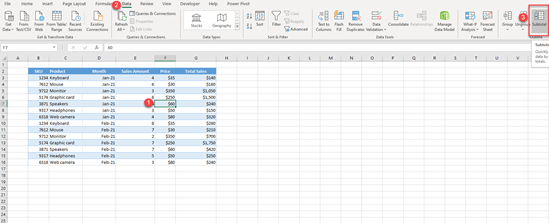The pop-up screen for Subtotal appears, and here we can specify how to group data. In the first section (At each change in:) we need to set Month, as we want to add a subtotal for each month. In the Add subtotal to: section, we need to check Total Sales, as we want to sum the Total Sales column (this is checked by default).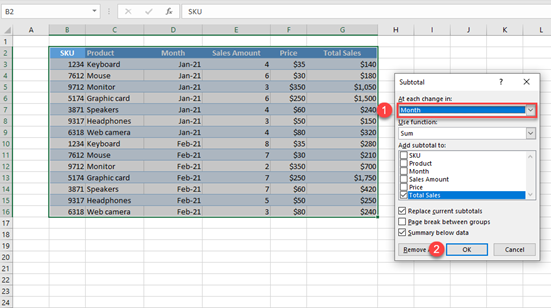We get the subtotal for each month (Jan-2021 and Feb-2021) as well as a Grand Total for the whole column.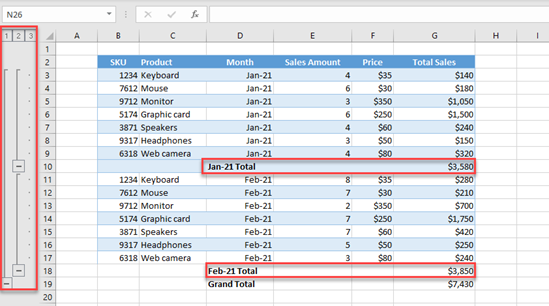We also have the data grouped by month now, so we can expand or collapse data by month.

## Remove Subtotal Rows

If we want to remove subtotal rows, we need to go again to the Data tab and Subtotal, and in the pop-up screen click on Remove All. This will remove all groupings and subtotals.